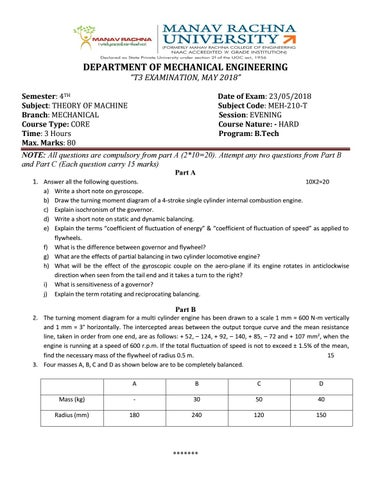DEPARTMENT OF MECHANICAL ENGINEERING “T3 EXAMINATION, MAY 2018” Semester: 4TH Subject: THEORY OF MACHINE Branch: MECHANICAL Course Type: CORE Time: 3 Hours Max. Marks: 80

Date of Exam: 23/05/2018 Subject Code: MEH-210-T Session: EVENING Course Nature: - HARD Program: B.Tech

NOTE: All questions are compulsory from part A (2*10=20). Attempt any two questions from Part B and Part C (Each question carry 15 marks) Part A 1. Answer all the following questions.

10X2=20

a) b) c) d) e)

Write a short note on gyroscope. Draw the turning moment diagram of a 4-stroke single cylinder internal combustion engine. Explain isochronism of the governor. Write a short note on static and dynamic balancing. Explain the terms “coefficient of fluctuation of energy” & “coefficient of fluctuation of speed” as applied to flywheels. f) What is the difference between governor and flywheel? g) What are the effects of partial balancing in two cylinder locomotive engine? h) What will be the effect of the gyroscopic couple on the aero-plane if its engine rotates in anticlockwise direction when seen from the tail end and it takes a turn to the right? i) What is sensitiveness of a governor? j) Explain the term rotating and reciprocating balancing.

Part B 2. The turning moment diagram for a multi cylinder engine has been drawn to a scale 1 mm = 600 N-m vertically and 1 mm = 3° horizontally. The intercepted areas between the output torque curve and the mean resistance line, taken in order from one end, are as follows: + 52, – 124, + 92, – 140, + 85, – 72 and + 107 mm2, when the engine is running at a speed of 600 r.p.m. If the total fluctuation of speed is not to exceed ± 1.5% of the mean, find the necessary mass of the flywheel of radius 0.5 m. 15 3. Four masses A, B, C and D as shown below are to be completely balanced. A

B

C

D

Mass (kg)

-

30

50

40

180

240

120

150

*******

The planes containing masses B and C are 300 mm apart. The angle between planes containing B and C is 90Â°. B and C make angles of 210Â° and 120Â° respectively with D in the same sense. Find the magnitude and the angular position of mass A; and The position of planes A and D. 15 4. Derive an expression for primary and secondary unbalance force in case of In-line engine. 15

Part C 5. A rear engine automobile is travelling along a track of 100 meters mean radius. Each of the four road wheels has a moment of inertia of 2.5 kg-m2 and an effective diameter of 0.6 m. The rotating parts of the engine have a moment of inertia of 1.2 kg-m2. The engine axis is parallel to the rear axle and the crankshaft rotates in the same sense as the road wheels. The ratio of engine speed to back axle speed is 3:1. The automobile has a mass of 1600 kg and has its centre of gravity 0.5 m above road level. The width of the track of the vehicle is 1.5 m. Determine the limiting speed of the vehicle around the curve for all four wheels to maintain contact with the road surface. Assume that the road surface is not cambered and centre of gravity of the automobile lies centrally with respect to the four wheels. 15 6. Explain the terms and derive the expressions for height, effort and power of a porter governor. 15 7. A Porter governor has equal arms each 250 mm long and pivoted on the axis of rotation. Each ball has a mass of 5 kg and the mass of the central load on the sleeve is 25 kg. The radius of rotation of the ball is 150 mm when the governor begins to lift and 200 mm when the governor is at maximum speed. Find the range of speed, sleeve lift, governor effort and power of the governor. 15

*******